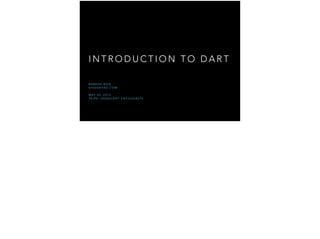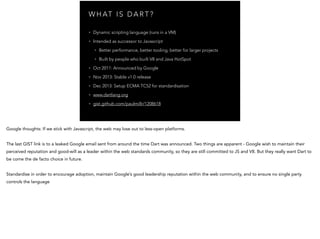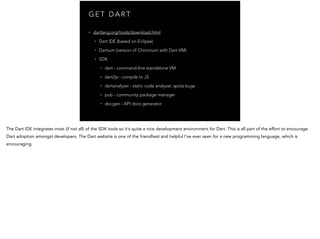Successfully reported this slideshow.

# Introduction to Dart

3

Share×
1 of 46
1 of 46

# Introduction to Dart

3

Share

Introduction to Google's Dart Programming language, with a brief overview of the major language features.

Introduction to Google's Dart Programming language, with a brief overview of the major language features.

## More Related Content

### Related Books

Free with a 14 day trial from Scribd

See all

### Related Audiobooks

Free with a 14 day trial from Scribd

See all

### Introduction to Dart

1. 1. I N T R O D U C T I O N T O D A R T R A M E S H N A I R H I D D E N TA O . C O M ! M A Y 3 0 , 2 0 1 4 TA I P E I J A VA S C R I P T E N T H U S I A S T S
2. 2. W H AT I S D A R T ? • Dynamic scripting language (runs in a VM) • lntended as successor to Javascript • Better performance, better tooling, better for larger projects • Built by people who built V8 and Java HotSpot • Oct 2011: Announced by Google • Nov 2013: Stable v1.0 release • Dec 2013: Setup ECMA TC52 for standardisation • www.dartlang.org • gist.github.com/paulmillr/1208618
3. 3. G E T D A R T • dartlang.org/tools/download.html • Dart IDE (based on Eclipse) • Dartium (version of Chromium with Dart VM) • SDK • dart - command-line standalone VM • dart2js - compile to JS • dartanalyzer - static code analyzer, spots bugs • pub - community package manager • docgen - API docs generator
4. 4. T H E B A S I C S L E T ’ S B E G I N …
5. 5. A S I M P L E P R O G R A M // short-hand function notation + parameter type and return types double show(double v) => v * 2.0; ! // program entry point, with void return type void main() { // dynamic type var a = 2.222; print("Result: \${show(a)}"); /* Result: 4.444 */ ! // everything is an object assert(a is Object); ! // converting to other types print(a.toInt() is int); /* true*/ print(a.toString() is String); /* true */ print(double.parse(a.toStringAsFixed(1))); /* 2.2 */ }
6. 6. O P T I O N A L T Y P E A N N O TAT I O N S • Help your IDE and other tools help you • Let the “static checker” warn you about potential problems, though code will still compile • Can help improve performance when compiling to JS • At runtime type annotations are only used if the Dart VM is in “Checked” mode… // either is ok int a = 12; var b = 12;
7. 7. D A R T R U N T I M E M O D E S • Checked mode • VM inserts type assertion checks at runtime • Will throw exception if types don’t match up: • If expecting type A then you can use an item which is type A or any of A’s subtypes. • Will throw exception if an assert() statement fails • Production mode (default) • Ignores all type annotations • Better performance because no type checks needed • Ignores all assert() statements
8. 8. F U N C T I O N D E F I N I T I O N var loudify = (msg) => '!!! \${msg.toUpperCase()} !!!'; print(loudify('hello')); /* !!! HELLO !!! */ void show({firstName: 'Foo', lastName: 'Bar'}) { print("\${firstName} \${lastName}"); } ! show('Mark'); /* throws Exception */ show('Mark', 'Twain'); /* throws Exception */ show(firstName: 'Mark'); /* Mark Bar */ show(lastName: 'Twain'); /* Foo Twain */
9. 9. T Y P E D E F S typedef int Compare(int a, int b); ! int comp1(int a, int b) => a - b; ! int comp2(bool a, int b) => a - b; ! String run(Compare sortFunction) => 'ok'; ! void main() { print(comp1 is Function); /* true */ print(comp1 is Compare); /* true */ ! print(run(comp1)); /* ok */ ! print(run(comp2)); /* throws TypeError */ }
10. 10. A N D T H E R E S T… • Closures - similar to Javascript • Operators - Arithmetic, logic, assignment, bitwise, comparison, etc. • Collections - lists (like Javascript arrays), sets, maps • Control flow - if-else, for/do-while, switch-case • Exceptions - throw, catch, finally • Reflections API • Typed data, SIMD, etc.
11. 11. O B J E C T O R I E N T E D P R O G R A M M I N G A R C H I T E C T U R E
12. 12. B A S I C C L A S S E S class StrRep { String str; String finalStr; num count; // constructor with initializer list StrRep(this.str, this.count); ! build() => finalStr = str.trim().toUpperCase() + count.toString(); void display() => print(this.finalStr); } ! void main() { // use the cascade operator (..) new StrRep('NaN ', 3) ..build() ..display(); /* NAN3 */ }
13. 13. G E T T E R S A N D S E T T E R S class Box { num _top; ! Box(this._top); num get top => _top; set top(num value) => _top = value * 2; } ! main() { var box = new Box(5); print(box.top); /* 5 */ box.top = 5; print(box.top); /* 10 */ }
14. 14. FA C T O RY / S I N G L E T O N class Store { var data; static Store _cache = null; // visible constructor factory Store() { if (null == _cache) { _cache = new Store._internal(); } return _cache; } // hidden constructor Store._internal(); } ! void main() { new Store().data = 'secret stuff'; var b = new Store(); print(b.data); /* secret stuff */ }
15. 15. O P E R AT O R O V E R L O A D class Vector { final int x; final int y; Vector(this.x, this.y); ! // Override + (a + b). Vector operator +(Vector v) { return new Vector(x + v.x, y + v.y); } } ! main() { // final = cannot modify this variable after this final v = new Vector(2,3); final w = new Vector(2,2); ! assert((v+w).x == 4 && (v+w).y == 5); // v+w == (4,5) }
16. 16. S I M P L E I N H E R I TA N C E class Shape { void draw() => print('shape'); } ! class Rectangle extends Shape {} ! class Square extends Rectangle { void draw() => print('square'); } ! void main() { new Rectangle().draw(); /* shape */ new Square().draw(); /* square */ }
17. 17. G E N E R I C S class Cache<T> { Map<String,T> _m; Cache() { _m = new Map<String, T>(); } T get(String key) => _m[key]; set(String key, T value) => _m[key] = value; } ! void main() { var c = new Cache<String>(); c.set('test', 'value'); print(c.get('test') == 'value'); c.set('test', 43); // fails in checked mode } Love this? read C++ Template Metaprogramming
18. 18. M O R E O O P… • Implicit Interfaces - if you implement a class then it becomes a specification • Abstract classes - if you don’t want the parent class to ever be instantiated make it abstract • Mixins - if you want to inherit from more than one class this helps
19. 19. L I B R A R I E S A N D PA C K A G E S S H A R I N G C O D E
20. 20. L I B R A R I E S NOTE: Identifiers that start with underscore (_) are not visible outside the library library calc; // optional ! int _add(int x, int y) => x + y; ! int sum3(int x, int y, int z) => _add(_add(x,y), z); import './calc.dart'; ! void main() { print(sum3(11, 12, 13)); /* 36 */ print(_add(11, 12)); /* throws NoSuchMethodError */ }
21. 21. B U I LT- I N L I B R A R I E S // only show certain items import "dart:math" as Math show PI; ! // hide certain items import "dart:collection" as Collection hide LinkedList; ! void main() { print(Math.PI); /* 3.14 */ print(Math.E); /* throws exception */ new Collection.LinkedList(); /* throws exception */ } • dart:core is always automatically imported • More at: https://api.dartlang.org/apidocs/channels/ stable/dartdoc-viewer/home
22. 22. C O M M U N I T Y L I B R A R I E S name: example description: A sample command-line application dependencies: quiver: any • pub.dartlang.org • Command-line package manager: pub \$ p u b g e t ! Resolving dependencies... Got dependencies! • Step 1 - specify dependencies in pubspec.yaml: • Step 2 - install dependencies:
23. 23. A S Y N C H R O N O U S P R O G R A M M I N G A L L A T O N C E …
24. 24. T H E “ G A M E ” L O O P
25. 25. A D D I N G A M I C R O TA S K • Can directly add to the microtask queue • Use scheduleMicrotask(fn) • Will ensure your task runs before the next event queue item ! • BUT this delays event processing • Use the event queue (i.e. a Future) when possible.
26. 26. F U T U R E S • Known as “Promises” in Javascript • Futures get added to the event queue • API can wrap sync methods, wait for multiple Futures, etc. import “dart:async"; ! Future result = costlyQuery(); ! result .then((value) => expensiveWork(value)) .then((value) => lengthyComputation(value)) .then((value) => print(value)) .catchError((exception) => print('DOH!')) .whenComplete(closeConnection); // may return a Future
27. 27. S T R E A M S • Produce one or more events that listeners receive • Two types • Broadcast - multiple listeners, doesn’t wait for listeners before sending • Single-subscription - one listener, queues events and awaits a listener • Can be paused (will buffer events until unpaused) • Can be proxied and transformed (e.g. music processing) • Auto-unsubscribe listeners when ‘done’ event sent
28. 28. E X A M P L E S T R E A M import 'dart:async'; ! main() { var data = [1,2,3,4,5]; // some sample data var stream = new Stream.fromIterable(data); ! // subscribe to the streams events stream.listen((value) { // print("Received: \$value"); // onData handler }); // }
29. 29. I S O L AT E S • Isolate - light-weight unit of concurrency • Each has its own memory and “thread of control” • Uses message passing to communicate with others • Main app always runs within its own isolate • Standalone Dart VM always runs isolates in parallel, using all available CPU cores (yay!) • Browser Dart VM may use web workers, thought not every browser implementation may do this
30. 30. // alice.dart ! import "dart:async"; import "dart:isolate"; ! void main() { var response = new ReceivePort(); Future<Isolate> remote = Isolate.spawnUri(Uri.parse("bob.dart"), ["foo"], response.sendPort); remote.then((_) => response.first) .then((msg) { print("received: \$msg"); }); } // bob.dart ! import "dart:isolate"; ! void main(List<String> args, SendPort replyTo) { replyTo.send(args); }
31. 31. O T H E R A S Y N C S T U F F… • Futures - delaying execution, waiting for multiple • Streams - testing event before handling it • Zones - asynchronous dynamic extents • Timers • I/O
32. 32. B U I L D I N G W E B A P P S H T M L + J A VA S C R I P T
33. 33. E M B E D I N H T M L <!-- For browsers with the Dart VM --> <script type='application/dart' src='app.dart'></script> ! <!-- For browsers without Dart VM, will fetch app.dart.js --> <script src="packages/browser/dart.js"></script> 1. Compile into JS \$ d a r t 2 j s - o a p p . d a r t . j s a p p . d a r t 2. Add to end of HTML body • main() invoked after DOM content is loaded. • One Dart script per page. Do not use inline scripts, async/ defer, or rely on injection of code or ordering of scripts
34. 34. K N O W Y O U R B L O AT void main() { print('Hello, World!'); } • dart2js output: 14,334 bytes • Browser package (dart.js): 1,270 bytes ! • Total: 15,645 bytes (~15 KB) • And don’t forget app startup time • Dart source: 41 bytes
35. 35. C A L L I N T O J AVA S C R I P T import 'dart:js'; ! void main() { var p1 = new JsObject(context['Point'], [5, 1]); ! p1['x'] = 100; print(p1['x']); // Prints 100. var p2 = new JsObject(context['Point'], [1, -2]); print(p1.callMethod('distanceFrom', [p2])); } var Point = function(x, y) { this.x = x; this.y = y; this.distanceFrom = function(otherPoint) { return Math.sqrt(Math.pow(otherPoint.x - this.x, 2) + Math.pow(otherPoint.y - this.y, 2)); }; };
36. 36. D O M I N T E R A C T I O N S import "dart:html"; ! void main() { // returns List<Element> var buttons = document.querySelectorAll('.clearfix'); // attributes = Map<String,String> buttons.attributes.remove('name'); var newElem = new ButtonElement() ..id = 'mozillaBtn' ..classes.add('mozilla') // Set<String> ..text = 'Mozilla'; document.nodes.add(newElem); // events are streams newElem.onClick.listen( (event) => print('click!') ); }
37. 37. C H E C K O U T… • Polymer - web components • AngularDart (port of Angular.js) • JSON serialization/de-serialization • HttpRequest (like XMLHttpRequest) • IndexedDB • SVG • Web audio, WebGL
38. 38. P E R F O R M A N C E V S J AVA S C R I P T D A R T …
39. 39. J AVA S C R I P T C O M PA R I S O N • https://www.dartlang.org/performance/ • Dart always faster than Javascript • dart2js output mostly slower than Javascript • http://www.techempower.com/benchmarks/ • Server-side Dart still mostly slower than Node.js But why does Dart perform better?
40. 40. W H Y D A R T P E R F O R M S B E T T E R • Simpler object model • Straightforward language semantics • Fewer special corner cases to worry about • e.g. Can’t modify Object prototype at runtime • More predictable performance • Better memory utilisation • Less generated code
41. 41. F I N D O U T M O R E … • https://www.youtube.com/watch?v=huawCRlo9H4 • Google I/O 2013 talk on Dart and JS VM performance • Also talks about work needed to ship Dart VM with Chrome browser
42. 42. PA R A L L E L P R O C E S S I N G • SIMD • “Single Instruction Multiple Data” • Float32x4, Int32x4 • Operate on numbers in parallel • Efficient CPU usage • Potential speedup of 400% over non-parallel computation • Useful for 3D graphics, audio processing, etc.
43. 43. T H E F U T U R E L O O K T O …
44. 44. W H O I S U S I N G I T N O W ? • drone.io - Continuous integration server • worktrail.net - time tracking app • Spark - Chrome app-based IDE • anionu.com - cloud security • …many more ! • https://www.dartlang.org/community/who-uses-dart.html
45. 45. B R O W S E R S U P P O R T • Chrome: Coming soon, need to implement new DOM-to-VM memory management model first. • Firefox: "Most browsers have no reason to include Dart because doing so would not only be extremely difficult for engineers to accomplish in each major browser, it would also result in a quality hit,” • Safari: ”Two runtimes sharing the DOM adds both bug habitat and a performance tax” • IE: "In practice, JavaScript performance is not the bottleneck in real-world performance”. • Microsoft are banking on TypeScript, a language which translates to Javascript
46. 46. @ H I D D E N TA O That’s all folks!